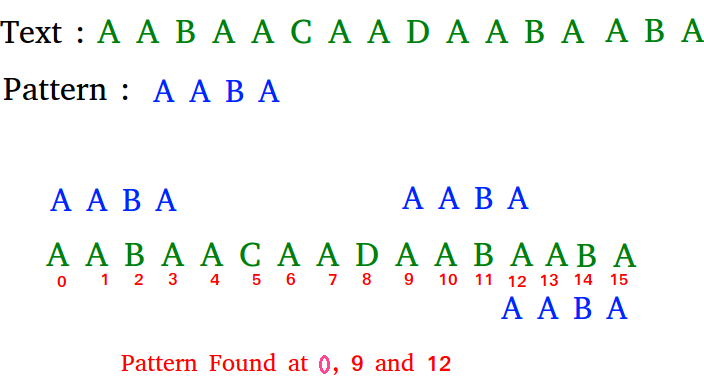GFG App
Open AppBrowser
Continue

# Java Program for Naive algorithm for Pattern Searching

Given a text txt[0..n-1] and a pattern pat[0..m-1], write a function search(char pat[], char txt[]) that prints all occurrences of pat[] in txt[]. You may assume that n > m.

Examples:

```Input:  txt[] = "THIS IS A TEST TEXT"
pat[] = "TEST"
Output: Pattern found at index 10

pat[] =  "AABA"
Output: Pattern found at index 0
Pattern found at index 9
Pattern found at index 12```

Pattern searching is an important problem in computer science. When we do search for a string in notepad/word file or browser or database, pattern searching algorithms are used to show the search results.

## Java

 `// Java program for Naive Pattern Searching` `public` `class` `NaiveSearch {`   `    ``public` `static` `void` `search(String txt, String pat)` `    ``{` `        ``int` `M = pat.length();` `        ``int` `N = txt.length();`   `        ``/* A loop to slide pat one by one */` `        ``for` `(``int` `i = ``0``; i <= N - M; i++) {`   `            ``int` `j;`   `            ``/* For current index i, check for pattern ` `              ``match */` `            ``for` `(j = ``0``; j < M; j++)` `                ``if` `(txt.charAt(i + j) != pat.charAt(j))` `                    ``break``;`   `            ``if` `(j == M) ``// if pat[0...M-1] = txt[i, i+1, ...i+M-1]` `                ``System.out.println(``"Pattern found at index "` `+ i);` `        ``}` `    ``}`   `    ``public` `static` `void` `main(String[] args)` `    ``{` `        ``String txt = ``"AABAACAADAABAAABAA"``;` `        ``String pat = ``"AABA"``;` `        ``search(txt, pat);` `    ``}` `}` `// This code is contributed by Harikishore`

Output:

```Pattern found at index 0
Pattern found at index 9
Pattern found at index 13
```

Time Complexity: O(M * (N – M + 1)), where M and N represents the length of the given strings.
Auxiliary Space: O(1), no extra space is required, so it is a constant.

Please refer complete article on Naive algorithm for Pattern Searching for more details!

My Personal Notes arrow_drop_up